# Analytical Reasoning (Test 5)

## Cat Entrance Exams : Data Interpretation And Logical Reasoning

| Home | | Cat Entrance Exams | | Data Interpretation And Logical Reasoning | | Analytical Reasoning |Analytical Reasoning
| Analytical Reasoning |
Q.1
In the adjoining figure, if the centres of all the circles are joined by horizontal and vertical lines, then find the number of squares that can be formed.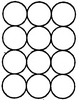A. 6
B. 7
C. 8
D. 1
Explaination / Solution:

The figure may be labelled as shown.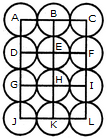We shall join the centres of all the circles by horizontal and vertical lines and then label the resulting figure as shown.

The simplest squares are ABED, BCFE, DEHG, EFIH, GHKJ and HILK i.e. 6 in number.

The squares composed of four simple squares are ACIG and DFLJ i.e. 2 in number.

Thus, 6 + 2 = 8 squares will be formed.

Workspace
Report
Q.2

Count the number of triangles and squares in the given figure.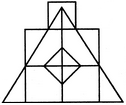A. 21 triangles, 7 squares
B. 18 triangles, 8 squares
C. 22 triangles, 7 squares
D. 20 triangles, 8 squares
Explaination / Solution:

The figure may be labelled as shown.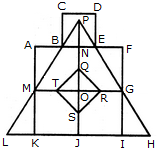Triangles :

The simplest triangles are BPN, PNE, ABM, EFG, MLK, GHI, QRO, RSO, STO and QTO i.e. 10 in number.

The triangles composed of two components each are BPE, TQR, QRS, RST and STQ i.e. 5 in number.

The triangles composed of three components each are MPO and GPO i.e. 2 in number.

The triangles composed of six components each are LPJ, HPJ and MPG i.e. 3 in number.

There is only one triangle LPH composed of twelve components.

Total number of triangles in the figure = 10 + 5 - 2 + 3 + 1 = 21.

Squares :

The squares composed of two components each are KJOM and JIGQ i.e. 2 in number.

The squares composed of three components each are ANOM, NFGO and CDEB i.e.3 in number.

There is only one square i.e. QRST composed of four components.

There is only one square i.e. AFIK composed of ten components.

Total number of squares in the figure = 2 + 3 + 1 + 1 = 7.

Workspace
Report
Q.3

Count the number of squares in the given figure.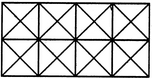A. 11
B. 21
C. 24
D. 26
Explaination / Solution:

The figure may be labelled as shown.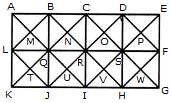The squares composed of two components each are BNQM, CORN, DPSO, MQTL, NRUQ, OSVR, PFWS, QUJT, RVIU and SWHV i.e. 10 in number.

The squares composed of four components each are ABQL, BCRQ, CDSR, DEFS, LQJK, QRIJ, RSHI and SFGH i.e. 8 in number.

The squares composed of eight components each are BRJL, CSIQ and DFHR i.e. 3 in number.

The squares composed of sixteen components each are ACIK, BDHJ and CEGI i.e. 3 in number.

Thus, there are 10 + 8 + 3 + 3 = 24 squares in the figure.

Workspace
Report
Q.4

How many triangles and parallelograms are there in the following figure?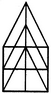A. 21, 17
B. 19, 13
C. 21, 15
D. 19, 17
Explaination / Solution:

The figure may be labelled as shown.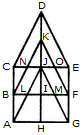Triangles:

The simplest triangles are KJN, KJO, CNB, OEF, JIL, JIM, BLA and MFG i.e. 8 in number.

The triangles composed of two components each are CDJ, EDJ, NKO, JLM, JAH and JGH i.e. 6 in number.

The triangles composed of three components each are BKI, FKI, CJA and EJG i.e. 4 in number.

The triangles composed of four components each are CDE and AJG i.e. 2 in number.

The only triangle composed of six components is BKF.

Thus, there are 8 + 6 + 4 + 2 + 1 = 21 triangles in the given figure.

Parallelograms :

The simplest parallelograms are NJLB and JOFM i.e. 2 in number.

The parallelograms composed of two components each are CDKB, DEFK, BIHA and IFGH i.e.4 in number.

The parallelograms composed of three components each are BKJA, KFGJ, CJIB and JEFI i.e.4 in number.

There is only one parallelogram i.e. BFGA composed of four components.

The parallelograms composed of five components each are CDJA, DEGJ, CJHA and JEGH i.e.4 in number.

The only parallelogram composed of six components is CEFB.

The only parallelogram composed of ten components is CEGA.

Thus, there are 2 + 4 + 4 + 1 + 4+ 1 + 1 = 17 parallelograms in the given figure.

(Here note that the squares and rectangles are also counted amongst the parallelograms).

Workspace
Report
Q.5

Count the number of squares in the given figure.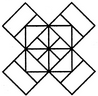A. 22
B. 20
C. 18
D. 14
Explaination / Solution:

The figure may be labelled as shown.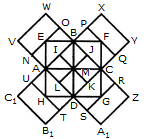The squares composed of two components each are BJMI, CKMJ, DLMK and AIML i.e. 4 in number.

The squares composed of three components each are EBMA, BFCM, MCGD and AMDH i.e. 4 in number.

The squares composed of four components each are VWBA, XYCB, ZA1DC and B1C1AD i.e. 4 in number.

The squares composed of seven components each are NOJL, PQKI, RSLJ and TUIK i.e. 4 in number.

There is only one square i.e. ABCD composed of eight components.

There is only one square i.e. EFGH composed of twelve components.

Total number of squares in the figure = 4 + 4 + 4 + 4 + 1 + 1 = 18.

Workspace
Report
Q.6

Count the number of parallelogram in the given figure.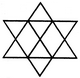A. 8
B. 11
C. 12
D. 15
Explaination / Solution:

The figure may be labelled as shown.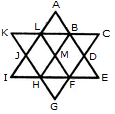The simplest parallelograms are LMHJ and BDFM i.e. 2 in number. The parallelograms composed of two components each are ABML and MFGH i.e. 2 in number.

The parallelograms composed of three components each are LBHI, LBEF, BDGH, DFLA, BCFH, KLFH, A6HJ and LFGJ i.e. 8 in number.

The parallelograms composed of six components each are LCFI, KBEH and ADGJ i.e. 3 in number.

Total number of parallelograms in the figure = 2 + 2 + 8 + 3 = 15.

Workspace
Report
Q.7

Count the number of convex pentagons in the adjoining figure.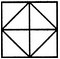A. 16
B. 12
C. 8
D. 4
Explaination / Solution:

A convex pentagon has no angles pointing inwards. More precisely, no internal angles can be more than 180°.

The figure may be labelled as shown.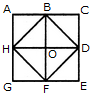The pentagons in the figure, are ABDFH, CDFHB, EFHBD, GHBDF, ACDFG, CEFHA, EGHBC, GABDE, BDEGH, DFGAB, FHACD and HBCEF. Clearly, these are 12 in number.

Workspace
Report
Q.8

Count the number of rectangles in the given figure.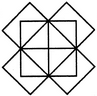A. 20
B. 18
C. 16
D. 15
Explaination / Solution:

The figure may be labelled as shown.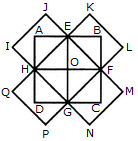The rectangles composed of two components each are HIJE, EKJ,F, FMNG, GPQH, AEOH, EBFO, OFCG and HOGD i.e. 8 in number.

The rectangles composed of four components each are ABFH, BCGE, CDHF, DAEG and EFGH i.e. 5 in number.

The rectangles composed of six components each are IJFG, KLGH, MNHE and PQEF i.e. 4 in number.

The rectangles composed of eight components each are IJMN, KLPQ and ABCD i.e. 3 in number.

Thus, there are 8 + 5 + 4 + 3 = 20 rectangles in the given figure.

(Here note that the squares are also counted amongst rectangles)

Workspace
Report
Q.9

Count the number of triangles and squares in the given figure.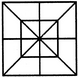A. 28 triangles, 10 squares
B. 28 triangles, 8 squares
C. 32 triangles, 10 squares
D. 32 triangles, 8 squares
Explaination / Solution:

The figure may be labelled as shown.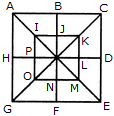Triangles :

The simplest triangles are IJQ, JKQ, KLQ, LMQ, MNQ, NOQ, OPQ and PIQ i.e. 8 in number. The triangles composed of two components each are ABQ, BCQ, CDQ, DEQ, EFQ, FGQ, GHQ, HAQ, IKQ, KMQ, MOQ and OIQ i.e. 12 in number.

The triangles composed of four components each are ACQ, CEQ, EGQ, GAQ, IKM, KMO, MOI and OIK i.e. 8 in number.

The triangles composed of eight components each are ACE, CEG, EGA and GAC i.e. 4 in number.

Total number of triangles in the figure = 8 + 12 + 8 + 4 = 32.

Squares :

The squares composed of two components each are IJQP, JKLQ, QLMN and PQNO i.e. 4 in number.

The squares composed of four components each are ABQH, BCDQ, QDEF and HQFG i.e. 4 in number.

There is only one square i.e. IKMO composed of eight components.

There is only one square i.e. ACEG composed of sixteen components.

Thus, there are 4 + 4 + 1 + 1= 10 squares in the given figure.

Workspace
Report
Q.10

Determine the number of rectangles and hexagons in the given figure.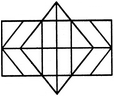A. 30, 5
B. 32, 3
C. 28, 5
D. 30, 3
Explaination / Solution:

The figure may be labelled as shown.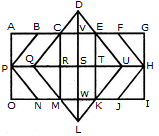Rectangles:

The simplest rectangles are CVSR, VETS, RSWM and STKW i.e. 4 in number.

The rectangles composed of two components each are CETR, VEKW, RTKM and CVWM i.e. 4 in number.

The rectangles composed of three components each are ACRP, PRMO, EGHT and THIK i.e. 4 in number.

The rectangles composed of four components each are CEKM, AVSP,PSWO, VGHS and SHIW i.e. 5 in number.

The rectangles composed of five components each are AETP, PTKO, CGHR and RHIM i.e. 4 in number.

The rectangles composed of six components each are ACMO and EGIK i.e. 2 in number.

The rectangles composed of eight components each are AGHP, PHIO, AVWO and VGIW i.e. 4 in number.

The rectangles composed of ten components each are AEKO and CGIM i.e. 2 in number.

AGIO is the only rectangle having sixteen components.

Total number of rectangles in the given figure

= 4 + 4 + 4 + 5 + 4 + 2 + 4 + 2 + 1 = 30.

Hexagons:

The hexagons in the given figure are CDEKLM, CEUKMQ, CFHJMQ, BEUKNP and BFHJNP.

So, there are 5 hexagons in the given figure.

Workspace
Report